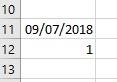Loading, please wait...## Commonly Used functions

1. Now- It is used to give the current date and current time.

Formula: “ =NOW() “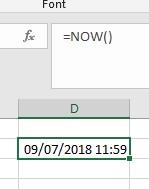1. Today – It gives the current date.

Formula: “ =TODAY()”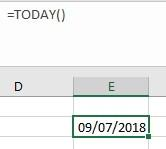1. Date – It takes three arguments namely “year” , “month” , “day”

Formula: “ =DATE(G2,H2,I2)”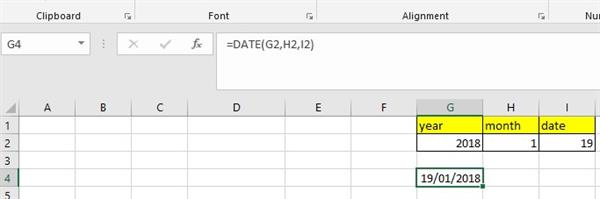• For extracting year, month, day from a given date we have predefined functions provided by excel.
• YEAR

It takes one argument as shown below.

OUTPUT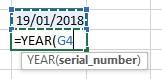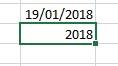• MONTH

It takes one argument as shown below

OUTPUT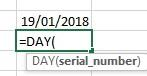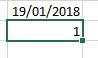• DAY

OUTPUT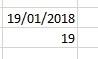1. TIME- It takes three arguments namely hour, minute, second.

It is used to get time if above mentioned parameters are given.

OUTPUT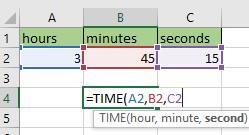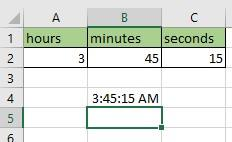1. For extracting hour, minute, seconds from a given time we have predefined functions provided by excel.
2. HOUR – It is used to extract hours from given time. It takes one argument.

OUTPUT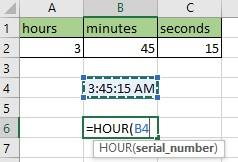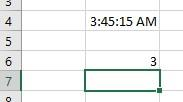• MINUTE- It is used to extract minutes from given time. It takes one argument.

OUTPUT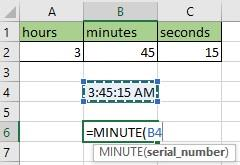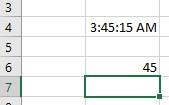• SECONDS- It is used to extract seconds from given time. It takes one argument.

OUTPUT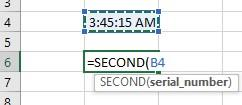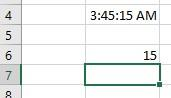1. NETWORKDAYS

It is used to get the total working days between start and end date excluding holidays.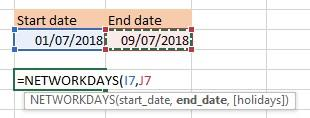OUTPUT

It gives the working days between start and end date excluding holidays.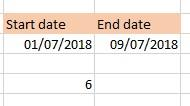1. WEEKDAY

It returns the week number corresponding to week of year. It returns numbers in range (1-54). It takes two arguments serial number and return type. You can choose the return type

Number (2) corresponds:

1 will represent Monday, 2 will represent Tuesday and so on till Sunday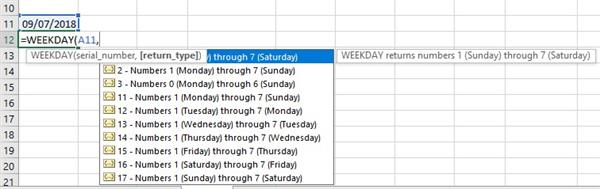OUTPUT